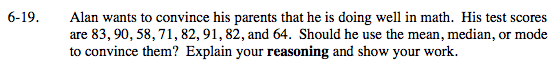### Home > MC2 > Chapter 6 > Lesson 6.1.2 > Problem6-19

6-19.Find the mean, the median, and the mode of Alan's test scores.

The mean is the average of Alan's test scores. The median is the middle number when the numbers are arranged numerically, or the average of the two middle numbers. The mode is the most reoccuring number in the set of data.

The mean is 77.625, the median is 82, and the mode is 82. Which answer(s) would convince his parents that Alan is doing well in math?

Either the median or the mode because those scores are higher.